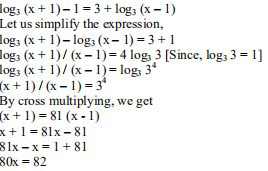# ML Aggarwal Solutions Class 9 Mathematics Solutions for Logarithms Exercise 9.2 in Chapter 9 - Logarithms

Solve for x:

\log _{3}(x+1)-1=3+\log _{3}(x-1)

Givenx = 82/80

= 41/40

= 1 1/40

Video transcript
"hey kids welcome to lido q a video i am vineeth your little tutor glad to bring you this question on your screen solve for x log x plus 1 base 3 minus 1 is equal to 3 plus log x minus 1 base 3 now we can write this as log x plus 1 base 3 minus log x minus 1 base 3 is equal to 3 plus 1 so this implies log x plus 1 by x minus 1 base 3 because log a minus log b is log a by b is equal to 4 right so we can say 4 4 log 3 base 3 because log 3 base 3 is 1 therefore log x plus 1 by x minus 1. base 3 is equal to log 3 to the power 4 base 3 so we can say x plus 1 by x minus 1 is equal to 81 so this implies x plus 1 is equal to 81x minus e2 so this implies atx is equal to 82 therefore x is equal to 82 by 80 or 41 by 40. isn't that easy guys if you still have it out please leave a comment below do like the video and subscribe to our channel i'll see you in our next video until then bye kids keep practicing"
Related Questions

Lido

Courses

Teachers

Book a Demo with us

Syllabus

Maths
CBSE
Maths
ICSE
Science
CBSE

Science
ICSE
English
CBSE
English
ICSE
Coding

Terms & Policies

Selina Question Bank

Maths
Physics
Biology

Allied Question Bank

Chemistry
Connect with us on social media!